# NCERT Solutions for Class 7 Maths Chapter 1: Integers

In this page we have NCERT Solutions for Class 7 Maths Chapter 1: Integers . Hope you like them and do not forget to like , social share and comment at the end of the page.

## Exercise 1.1

Question 1:
Following number line shows the temperature in degree celsius (°C) at different places on a particular day.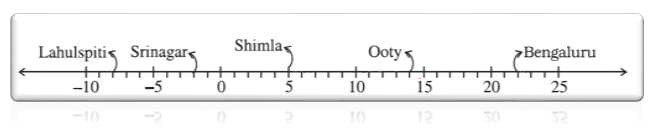(a) Observe this number line and write the temperature of the places marked on it.
(b) What is the temperature difference between the hottest and the coldest places among the above?
(c) What is the temperature difference between Lahulspiti and Srinagar?
(d) Can we say temperature of Srinagar and Shimla taken together is less than the temperature at Shimla? Is it also less than the temperature at Srinagar?
Solution
(a) By observing the given data, the temperatures of these cities are as follows.
 Lahulspiti −8°C Srinagar −2°C Shimla 5°C Ooty 14°C Bangalore 22°C

(b) Temperature at the hottest place, i.e., Bangalore = 22°C
Temperature at the coldest place, i.e., Lahulspiti = −8°C
Temperature difference = 22°C − (−8°C)
= 30°C
(c) Temperature at Lahulspiti = −8°C
Temperature at Srinagar = −2°C
Temperature difference = −2°C − (−8°C)
= 6°C
(d) Temperature at Srinagar = −2°C
Temperature at Shimla = 5°C
Temperature of Srinagar and Shimla taken together = −2°C + 5°C
= 3°C
3°C < 5°C
3°C < Temperature of Shimla Yes, the temperature of Srinagar and Shimla taken together is less than the temperature of Shimla. However, 3°C > −2°C
Hence, the temperature of Srinagar and Shimla taken together is not less than the temperature of Srinagar.
Question 2:
In a quiz, positive marks are given for correct answers and negative marks are given for incorrect answers. If jack’s scores in five successive rounds were 25, − 5, − 10, 15 and 10, what was his total at the end?
Solution
Jack’s scores in five successive rounds are 25, −5, −10, 15, and 10. Total score of Jack at the end will be the sum of these scores.
Therefore, Jack’s total score at the end = 25 − 5 − 10 + 15 + 10 = 35
Question 3:
At Srinagar temperature was − 5°C on Monday and then it dropped by 2°C on Tuesday.
What was the temperature of Srinagar on Tuesday? On Wednesday, it rose by 4°C. What was the temperature on this day?
Solution
Temperature on Monday = −5°C
Temperature on Tuesday = Temperature on Monday −2°C
= −5°C − 2°C = −7°C
Temperature on Wednesday = Temperature on Tuesday + 4°C
= −7°C + 4°C = −3°C
Therefore, the temperature on Tuesday and Wednesday was −7ºC and −3ºC respectively.
Question 4:
A plane is flying at the height of 5000 m above the sea level. At a particular point, it is exactly above a submarine floating 1200 m below the sea level. What is the vertical distance between them?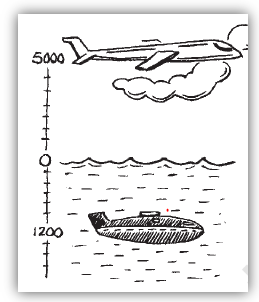Solution
Height of plane = 5000 m
Depth of submarine = −1200 m
Distance between plane and submarine = 5000 m − (−1200) m
= 5000 m + 1200 m = 6200 m
Question 5:
Mohan deposits Rs 2,000 in his bank account and withdraws Rs 1,642 from it, the next day. If withdrawal of amount from the account is represented by a negative integer, then how will you represent the amount deposited? Find the balance in Mohan’s account after the withdrawal.
Solution
Since the amount withdrawn is represented by a negative integer, the amount deposited will be represented by a positive integer.
Amount deposited = Rs 2000
Amount withdrawn = −Rs 1642
Balance in Mohan’s account = Money deposited + Money withdrawn
= 2000 + (−1642) = 2000 − 1642 = 358
Therefore, balance in Mohan’s account after withdrawal is Rs 358.
Question 6:
Rita goes 20 km towards east from a point A to the point B. From B, she moves 30 km towards west along the same road. If the distance towards east is represented by a positive integer then, how will you represent the distance travelled towards west? By which integer will you represent her final position from A?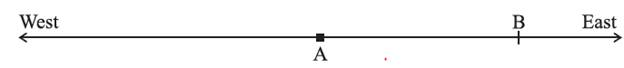Solution
Since the distance towards east is represented by a positive integer, the distance travelled towards west will be represented by a negative integer.
Distance travelled in east direction = 20 km
Distance travelled in west direction = −30 km
Distance travelled from A = 20 + (−30) = −10 km
Therefore, we will represent the distance travelled by Rita from point A by a negative integer, i.e., −10 km (i.e., Rita is now in west direction).
Question 7:
In a magic square each row, column and diagonal have the same sum. Check which of the following is a magic square.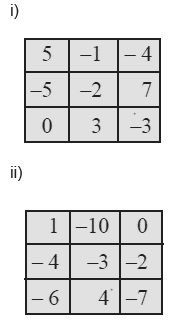Solution
It can be observed that in square (i), every row and column add up to give 0. However, the sum of one of its diagonals is not 0.
As − 4 − 2 = −6 ≠ 0,
Therefore, (i) is not a magic square.
Similarly, in square (ii), each row, column, and diagonal add up to give −9. Therefore,
(ii) is a magic square.
Question 8:
Verify a − (−b) = a + b for the following values of a and b.
(i) a = 21, b = 18
(ii) a = 118, b = 125
(iii) a = 75, b = 84
(iv) a = 28, b = 11
Solution
(i) a = 21, b = 18
LHS=a − (−b) = 21 − (−18) = 21 + 18 = 39
RHS=a + b = 21 + 18 = 39
Therefore,
a − (−b) = a + b = 39

(ii) a = 118, b = 125
LHS=a − (−b) = 118 − (−125) = 118 + 125 = 243
RHS=a + b = 118 + 125 = 243
Therefore,
a − (−b) = a + b = 243
(iii) a = 75, b = 84
LHS=a − (−b) = 75 − (−84) = 75 + 84 = 159
RHS=a + b = 75 + 84 = 159
Therefore,
a − (−b) = a + b = 159
(iv) a = 28, b = 11
LHS=a − (−b) = 28 − (−11) = 28 + 11 = 39
RHS=a + b = 28 + 11 = 39
Therefore,
a − (−b) = a + b = 39
Question 9:
Use the sign of >, < or = in the box to make the statements true.
(a) (– 8) + (– 4)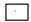(–8) – (– 4)
(b) (– 3) + 7 – (19)15 – 8 + (– 9)
(c) 23 – 41 + 1123 – 41 – 11
(d) 39 + (– 24) – (15)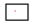36 + (– 52) – (– 36)
(e) – 231 + 79 + 51–399 + 159 + 81
Solution
a)  (– 8) + (– 4)(–8) – (– 4)
-8 -4  ___ -8 +4
-12  < -4
b)  (– 3) + 7 – (19)15 – 8 + (– 9)
-3 +7-19  __ 15-8-9
-15 < -2
c)  23 – 41 + 1123 – 41 – 11
-7  > -29
d)  39 + (– 24) – (15)36 + (– 52) – (– 36)
39 -24-15 ___ 36-52+36
0 < 20
e) – 231 + 79 + 51–399 + 159 + 81
-101 >159

Question 10:
A water tank has steps inside it. A monkey is sitting on the topmost step (i.e., the first step). The water level is at the ninth step.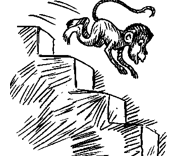(i) He jumps 3 steps down and then jumps back 2 steps up. In how many jumps will he reach the water level?
(ii) After drinking water, he wants to go back. For this, he jumps 4 steps up and then jumps back 2 steps down in every move. In how many jumps will he reach back the top step?
(iii) If the number of steps moved down is represented by negative integers and the number of steps moved up by positive integers, represent his moves in part (i) and (ii) by completing the following; (a) − 3 + 2 − … = − 8 (b) 4 − 2 + … = 8. In (a) the sum (− 8) represents going down by eight steps. So, what will the sum 8 in (b) represent?
Solution
Let the steps moved down be represented by positive integers and the steps moved up be represented by negative integers.
(i) Initially, the monkey was at step = 1
After 1st jump, the monkey will be at step = 1 + 3 = 4
After 2nd jump, the monkey will be at step = 4 + (−2) = 2
After 3rd jump, the monkey will be at step = 2 + 3 = 5
After 4th jump, the monkey will be at step = 5 + (−2) = 3
After 5th jump, the monkey will be at step = 3 + 3 = 6
After 6th jump, the monkey will be at step = 6 + (−2) = 4
After 7th jump, the monkey will be at step = 4 + 3 = 7
After 8th jump, the monkey will be at step = 7 + (−2) = 5
After 9th jump, the monkey will be at step = 5 + 3 = 8
After 10th jump, the monkey will be at step = 8 + (−2) = 6
After 11th jump, the monkey will be at step = 6 + 3 = 9
Clearly, the monkey will be at water level (i.e., 9th step) after 11 jumps.
(ii) Initially, the monkey was at step = 9
After 1st jump, the monkey will be at step = 9 + (−4) = 5
After 2nd jump, the monkey will be at step = 5 + 2 = 7
After 3rd jump, the monkey will be at step = 7 + (− 4) = 3
After 4th jump, the monkey will be at step = 3 + 2 = 5
After 5th jump, the monkey will be at step = 5 + (− 4) = 1
Clearly, the monkey will reach back at the top step after 5 jumps.
(iii) If steps moved down are represented by negative integers and steps moved up are represented by positive integers, then his moves will be as follows.
Moves in part (i)
− 3 + 2 − 3 + 2 − 3 + 2 − 3 + 2 − 3 + 2 − 3 = −8
Moves in part (ii)
4 − 2 + 4 − 2 + 4 = 8
Moves in part (ii) represent going up 8 steps.

## Exercise 1.2

Question 1:
Write down a pair of integers whose:
(a) sum is − 7
(b) difference is − 10
(c) sum is 0
Solution
(a) − 12 + (+5) = −7
(b) − 11 − (−1) = −10
(c)  7+ (−7) = 0
Question 2:
(a) Write a pair of negative integers whose difference gives 8.
(b) Write a negative integer and a positive integer whose sum is − 5.
(c) Write a negative integer and a positive integer whose difference is − 3.
Solution
(a) −3 − (−11) = 8
(b) −9 + 4 = −5
(c) −1 − (+2) = −3
Question 3:
In a quiz, team A scored − 40, 10, 0 and team B scored 10, 0 − 40 in three successive rounds. Which team scored more? Can we say that we can add integers in any order?
Solution
Team A scored − 40, 10, 0.
Total score = − 40 + 10 + 0
= −30
Team B scored 10, 0, −40.
Total score = 10 + 0 + (−40)
= −30
∴ The scores of both teams are equal.
Yes, we can add integers in any order. We had observed that the scores obtained by both teams in successive rounds were numerically equal but different in order. Yet, the total score of both teams were equal.
Question 4:
Fill in the blanks to make the following statements true:
(i) (− 5) + (− 8) = (− 8) + (…)
(ii) − 53 + … = − 53
(iii) 17 + … = 0
(iv) [13 + (− 12)] + (…) = 13 + [(− 12) + (− 7)]
(v) (− 4) + [15 + (− 3)] = [(− 4) + 15] + (….)
Solution
(i) (− 5) + (− 8) = (− 8) + ( -5)
(ii) − 53 + (0) = − 53
(iii) 17 + (-17) = 0
(iv) [13 + (− 12)] + (-7) = 13 + [(− 12) + (− 7)]
(v) (− 4) + [15 + (− 3)] = [(− 4) + 15] +(-3)

## Exercise 1.3

Question 1:
Find each of the following products:
(a) 3 × (− 1)
(b) (− 1) × 225
(c) (− 21) × (− 30)
(d) (− 316) × (− 1)
(e) (− 15) × 0 × (−18)
(f) (− 12) × (− 11) × (10)
(g) 9 × (− 3) × (− 6)
(h) (− 18) × (− 5) × (− 4)
(i) (− 1) × (−2) × (− 3) × 4
(j) (− 3) × (− 6) × (− 2) × (− 1)
Solution
(a) 3 × (−1) = −3
(b) (−1) × 225 = −225
(c) (−21) × (−30) = 630
(d) (−316) × (−1) = 316
(e) (−15) × 0 × (−18) = 0
(f) (−12) × (−11) × 10 = 1320
(g) 9 × (−3) × (−6) = 162
(h) (−18) × (−5) × (−4) = −360
(i) (−1) × (−2) × (−3) × 4 = −24
(j) (−3) × (−6) × (−2) × (−1) = 36
Question 2:
Verify the following:
(a) 18 × [7 + (–3)] = [18 × 7] + [18 × (–3)]
(b) (–21) × [(– 4) + (– 6)] = [(–21) × (– 4)] + [(–21) × (– 6)]

Solution
(a) L.H.S. = 18 × [7 + (− 3)] = 18 × [7 − 3] = 18 × 4 = 72
R.H.S. = [18 × 7] + [18 × (− 3)] = 126 + (− 54) = 72
Hence
18 × [7 + (–3)] = [18 × 7] + [18 × (–3)]

(b) L.H.S. = (−21) × [(−4) + (−6)] = (−21) × [− 4 − 6] = (−21) × [−10] = 210
R.H.S. = [(−21) × (−4)] + [(−21) × (−6)] = 84 + 126 = 210
Hence,
(–21) × [(– 4) + (– 6)] = [(–21) × (– 4)] + [(–21) × (– 6)]

Question 3:
(i) For any integer a, what is (− 1) × a equal to?
(ii) Determine the integer whose product with (− 1) is
(a) − 22 (b) 37 (c) 0
Solution
(i) (−1) × a = −a
ii)
a) 22 X (-1) =-22
b) (-37) X ( -1) =37
c) 0 X(-1) =0

Question 4:
Starting from (− 1) × 5, write various products showing some pattern to show (− 1) × (− 1) =1.
Solution
−1 × 5 = −5
−1 × 4 = −4 = − 5 + 1
−1 × 3 = −3 = − 4 + 1
−1 × 2 = −2 = − 3 + 1
−1 × 1 = −1 = − 2 + 1
−1 × 0 = 0 = − 1 + 1
Therefore, −1 × (−1) = 0 + 1 = 1
Question 5:
Find the product, using suitable properties:
(a) 26 × (− 48) + (− 48) × (− 36) (b) 8 × 53 × (−125)
(c) 15 × (− 25) × (− 4) × (− 10) (d) (− 41) × 102
(e) 625 × (− 35) + (− 625) × 65 (f) 7 × (50 − 2)
(g) (− 17) × (− 29) (h) (− 57) × (− 19) + 57
Solution
(a) 26 × (−48) + (−48) × (−36)
As multiplication is commutative for integers (b × a = a × b)
= (−48) × 26 + (−48) × (−36)
As per Distributivity of multiplication over addition (a × b + a × c) = a (b + c)
= (−48) [26 − 36]
= (−48) × (−10) = 480
(b) 8 ×53 × (−125) = 8 × [53 × (−125)]
As multiplication is commutative for integers (b × a = a × b)

= 8 × [(−125) × 53]
As per Associativity for Multiplication a × (b × c) = (a × b) × c
= [8 × (−125)] ×53
= [−1000] × 53 = −53000
(c) 15 × (−25) × (−4) × (−10)
= 15 × [(−25) × (−4)] × (−10)
= 15 ×  × (−10)
= 15 × (−1000) = −15000
(d) (−41) × 102
= (−41) × (100 + 2)
As per Distributivity of multiplication over addition (a × b + a × c) = a (b + c)

= (−41) × 100 + (−41) × 2
= − 4100 − 82 = −4182
(e) 625 × (−35) + (−625) × 65
As per Distributivity of multiplication over addition (a × b + a × c) = a (b + c)

= 625 × [(−35) + (−65)]
= 625 × [−100] = −62500
(f) 7 × (50 − 2)
As per Distributivity of multiplication over subtraction (a × b - a × c) = a (b - c)

= (7 × 50) − (7 × 2)= 350 − 14
= 336
(g) (−17) × (−29)
= (−17) × [−30 + 1]
As per Distributivity of multiplication over addition (a × b + a × c) = a (b + c)
= [(−17) × (−30)] + [(−17) × 1]
=  + [−17] = 493
(h) (−57) × (−19) + 57
= 57 × 19 + 57 × 1
As per Distributivity of multiplication over addition (a × b + a × c) = a (b + c)
= 57 [19 + 1]
= 57 × 20 = 1140
Question 6:
A certain freezing process requires that room temperature be lowered from 40°C at the rate of 5°C every hour. What will be the room temperature 10 hours after the process begins?
Solution
Initial temperature = 40°C
Change in temperature per hour = −5°C
Change in temperature after 10 hours = (−5) × 10 = −50°C
Final temperature = 40ºC + (−50ºC) = −10°C
Question 7:
In a class test containing 10 questions, 5 marks are awarded for every correct answer and (− 2) marks are awarded for every incorrect answer and 0 for questions not attempted.
(i) Mohan gets four correct and six incorrect answers. What is his score?
(ii) Reshma gets five correct answers and five incorrect answers, what is her score?
(iii) Heena gets two correct and five incorrect answers out of seven questions she attempts. What is her score?
Solution
(i) Marks given for 1 correct answer = 5
Marks given for 4 correct answers = 5 × 4 = 20
Marks given for 1 wrong answer = −2
Marks given for 6 wrong answers = −2 × 6 = −12
Score obtained by Mohan = 20 − 12 = 8
(ii) Marks given for 1 correct answer = 5
Marks given for 5 correct answers = 5 × 5 = 25
Marks given for 1 wrong answer = −2
Marks given for 5 wrong answers = −2 × 5 = −10
Score obtained by Reshma = 25 − 10 = 15
(iii) Similarly,
Marks given for 2 correct answers = 5 × 2 = 10
Marks given for 5 wrong answers = −2 × 5 = −10
Score obtained by Heena = 10 − 10 = 0
Question 8:
A cement company earns a profit of Rs 8 per bag of white cement sold and a loss of Rs 5 per bag of grey cement sold.
(a) The company sells 3, 000 bags of white cement and 5,000 bags of grey cement in a month. What is its profit or loss?
(b) What is the number of white cement bags it must sell to have neither profit nor loss, if the number of grey bags sold is 6,400 bags.
Solution
Profit is denoted by a positive integer and loss is denoted by a negative integer.
(a) Profit earned while selling 1 bag of white cement = Rs 8
Profit earned while selling 3000 bags of white cement = 8 × 3000
= 24000
Loss incurred while selling 1 bag of grey cement = −Rs 5
Loss incurred while selling 5000 bags of grey cement = −5 × 5000
= −25000
Total profit/loss earned = Profit + Loss
= 24000 + (−25000) = −1000
Therefore, a loss of Rs 1000 will be incurred by the company.
(b) Loss incurred while selling 1 bag of grey cement = −Rs 5
Loss incurred while selling 6400 bags of grey cement = (−5) × 6400
= −32000
Let the number of bags of white cement to be sold be x.
Profit earned while selling 1 bag of white cement = Rs 8
Profit earned while selling x bags of white cement = x × 8
= 8x
In condition of no profit no loss,
Profit earned + Loss incurred = 0
8x + (−32000) = 0
8x = 32000
x = 4000
Therefore, 4000 bags of white cement must be sold.
Question 9:
Replace the blank with an integer to make it a true statement.
(a) (–3) × _____ = 27
(b) 5 × _____ = –35
(c) _____ × (– 8) = –56
(d) _____ × (–12) = 132
Solution
a) (-3) X (-9) = 27
b) 5 X (-7) =-35
c) 7 X (-8) =-56
d) (-11) X (-12) =132

## Exercise 1.4

Question 1:
Evaluate each of the following:
(a) (–30) ÷ 10
(b) 50 ÷ (–5)
(c) (–36) ÷ (–9)
(d) (– 49) ÷ (49)
(e) 13 ÷ [(–2) + 1]
(f ) 0 ÷ (–12)
(g) (–31) ÷ [(–30) + (–1)]
(h) [(–36) ÷ 12] ÷ 3
(i) [(– 6) + 5)] ÷ [(–2) + 1]
Solution
(a) (−30) ÷ 10 = −3
(b) 50 ÷ (−5) = −10
(c) (−36) ÷ (−9) = 4
(d) (−49) ÷ 49 = −1
(e) 13 ÷ [−2 + 1] = 13 ÷ [−1] = −13
(f) 0 ÷ (−12) = 0
(g) (−31) ÷ [(−30) + (−1)] = (−31) ÷ (−31) = 1
(h) [(−36) ÷ 12] ÷ 3 = [−3] ÷ 3 = −1
(i) [− 6 + 5] ÷ [− 2 + 1] = (−1) ÷ (−1) = 1
Question 2:
Verify that a ÷ (b + c) ≠ (a ÷ b) + (a ÷ c) for each of the following values of a, b and c.
(a) a = 12, b = −4, c = 2
(b) a = (− 10), b = 1, c = 1
Solution
(a) a = 12, b = −4, c = 2
a ÷ (b + c) = 12 ÷ (− 4 + 2) = 12 ÷ (−2) = −6
(a ÷ b) + (a ÷ c) = [12 ÷ (−4)] + [12 ÷ 2] = −3 + 6 = 3
Hence, a ÷ (b + c) ≠ (a ÷ b) + (a ÷ c)
(b) a = −10, b = 1, c = 1
a ÷ (b + c) = (−10) ÷ (1 + 1) = (−10) ÷ 2 = −5
(a ÷ b) + (a ÷ c) = [(−10) ÷ 1] + [(−10) ÷ 1] = − 10 − 10 = −20
Hence, a ÷ (b + c) ≠ (a ÷ b) + (a ÷ c)
Question 3:
Fill in the blanks:
(a) 369 ÷ _____ = 369
(b) (–75) ÷ _____ = –1
(c) (–206) ÷ _____ = 1
(d) – 87 ÷ _____ = 87
(e) _____ ÷ 1 = – 87
(f) _____ ÷ 48 = –1
(g) 20 ÷ _____ = –2
(h) _____ ÷ (4) = –3
Solution
a) 369 ÷ (1) = 369
(b) (–75) ÷ (75) = –1
(c) (–206) ÷ (-1) = 1
(d) – 87 ÷ (-1) = 87
(e) -87 ÷ (1) = – 87
(f) -48 ÷ 48 = –1
(g) 20 ÷ -10 = –2
(h) -12 ÷ (4) = –3

Question 4:
Write five pairs of integers (a, b) such a ÷ b = − 3. One such pair is (6, − 2) because 6 ÷ (− 2) = (− 3).
Solution
(i) (18, −6)
Because 18 ÷ (−6) = −3
(ii) (−3, 1)
Because (−3) ÷ 1 = −3
(iii) (27, −9)
Because 27 ÷ (−9) = −3
(iv) (−33, 11)
Because (−33) ÷ 11 = −3
(v) (24, −8)
Because 24 ÷ (−8) = −3

Question 5:
The temperature at 12 noon was 10°C above zero. If it decreases at the rate of 2°C per hour until midnight, at what time would the temperature be 8°C below zero? What would be the temperature at mid-night?
Solution
Initial temperature i.e., at 12 noon = 10°C
Change in temperature per hour = −2°C
Temperature at 1:00 PM = 10ºC + (−2ºC) = 8ºC
Temperature at 2:00 PM = 8ºC + (−2ºC) = 6ºC
Temperature at 3:00 PM = 6ºC + (−2ºC) = 4ºC
Temperature at 4:00 PM = 4ºC + (−2ºC) = 2ºC
Temperature at 5:00 PM = 2ºC + (−2ºC) = 0ºC
Temperature at 6:00 PM = 0ºC + (−2ºC) = −2ºC
Temperature at 7:00 PM = −2ºC + (−2ºC) = −4ºC
Temperature at 8:00 PM = −4ºC + (−2ºC) = −6ºC
Temperature at 9:00 PM = −6ºC + (−2ºC) = −8ºC
Therefore, the temperature will be 8°C below zero at 9:00 PM.
It will take 12 hours to be midnight (i.e., 12:00 AM) after 12:00 noon.
Change in temperature in 12 hours = −2°C × 12 = −24ºC
At midnight, the temperature will be = 10 + (−24)
= −14°C
Therefore, the temperature at midnight will be 14ºC below 0.
Question 6:
In a class test (+ 3) marks are given for every correct answer and (−2) marks are given for every incorrect answer and no marks for not attempting any question. (i) Radhika scored 20 marks. If she has got 12 correct answers, how many questions has she attempted incorrectly? (ii) Mohini scores − 5 marks in this test, though she has got 7 correct answers. How many questions has she attempted incorrectly? (iii) Rakesh scores 18 marks by attempting 16 questions. How many questions has he attempted correctly and how many has he attempted incorrectly?
Solution
Marks obtained for 1 right answer = +3
Marks obtained for 1 wrong answer = −2
(i) Marks scored by Radhika = 20
Marks obtained for 12 correct answers = 12 × 3 = 36
Marks obtained for incorrect answers = Total score − Marks obtained for 12 correct answers
= 20 − 36 = −16
Marks obtained for 1 wrong answer = −2
Thus, number of incorrect answers = (−16) ÷(−2) = 8
Therefore, she attempted 8 questions wrongly.
(ii) Marks scored by Mohini = −5
Marks obtained for 7 correct answers = 7 × 3 = 21
Marks obtained for incorrect answers = Total score − Marks obtained for 12 correct answers
= − 5 − 21 = −26
Marks obtained for 1 wrong answer = −2
Thus, number of incorrect answers = (−26) ÷(−2) = 13
Therefore, she attempted 13 questions wrongly.
(iii) Total marks scored by Rakesh = 18
Number of questions attempted = 16
(Number of correct answers)(3) + (16 − Number of correct answers)(−2) = 18
(Number of correct answers)(3) + −32 + 2(Number of correct answers) = 18
(Number of correct answers)(5) + −32 = 18
(Number of correct answers)(5) = 18 + 32 = 50
Number of correct answers = 10
Therefore,
Number of incorrect answers = 16 − 10 = 6
Therefore,
Total number of correct and incorrect answers scored by Rakesh is 10 and 6 respectively.
Question 7:
An elevator descends into a mine shaft at the rate of 6 m/min. If the descent starts from 10 m above the ground level, how long will it take to reach − 350 m.
Solution
Distance descended is denoted by a negative integer.
Initial height = +10 m
Final depth = −350 m
Total distance to be descended by the elevator = (−350) − (+10) = −360 m
Time taken by the elevator to descend −6 m = 1 min
Thus, time taken by the elevator to descend −360 m = (−360) ÷ (−6)
= 60 minutes = 1 hour

### Similar type questions

Find:
a)4 × (– 8),
b)8 × (–2),
c) 3 × (–7),
d) 10 × (–1)
e) 15 × (–16)
(f) 21 × (–32)
(g) (– 42) × 12
(h) –55 × 15
i) (–1) × (–1)
j) (–1) × (–1) × (–1)
k) (–1) × (–1) × (–1) × (–1)
l) (–1) × (–1) × (–1) × (–1) × (–1)